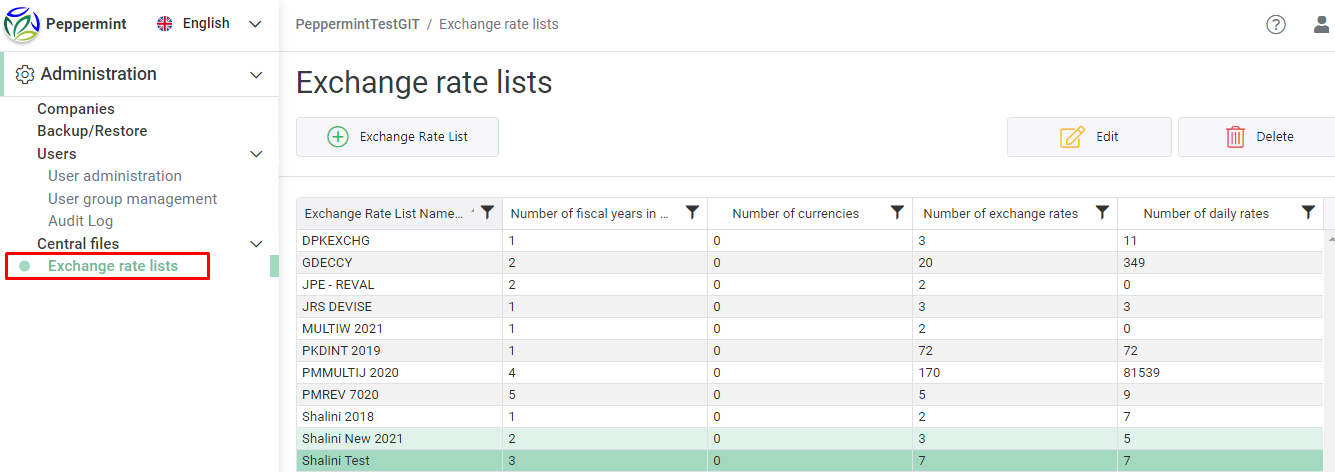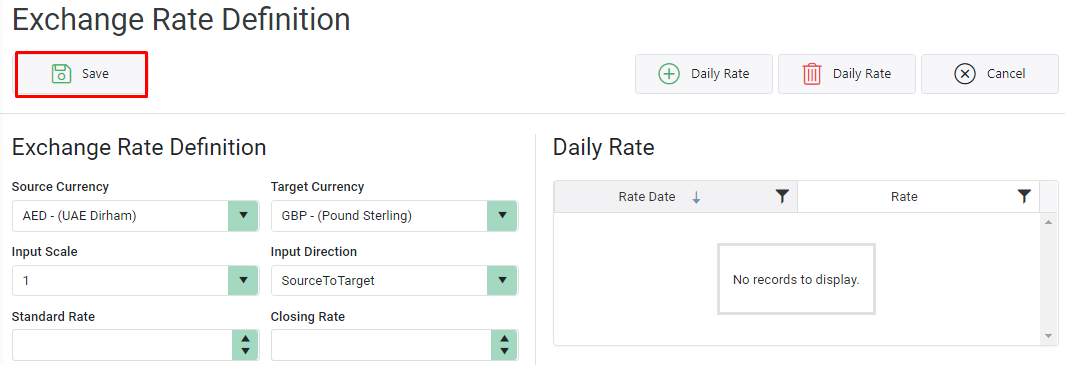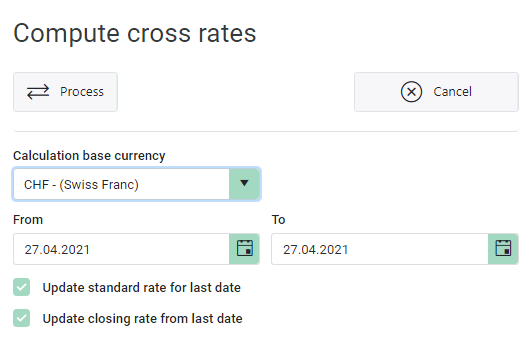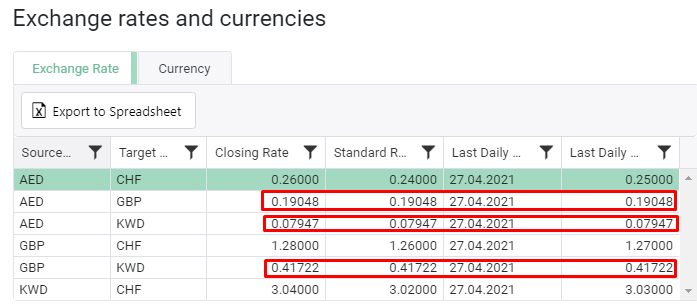“Compute Cross rates” button is to auto-calculate Cross exchange rates based on Base currency daily rates.

Let us understand the concept by below example,

Go to “Exchange rate lists” from Central files. Open relevant Exchange rate listSuppose we have below Exchange rates maintained in the Exchange rate list for base currency CHF“Compute cross rates” button will be able to calculate daily cross rates given base currency CHF for “AED <-> GBP”, “GBP <-> KWD” & “AED <-> KWD”.

Firstly, define Exchange rates for AED, GBP & KWD. Daily, Standard & Closing rates need not be defined.Now, click on “Compute cross rates” button.Give Base currency as CHF, Date range and Process it. Check below boxes if relevant:

Update standard rate for last date: Cross Rate of Last date will be filled as Standard rate

Update closing rate from last date: Cross Rate of Last date will be filled as Closing rateOnce processed, Cross rates will be successfully calculated for defined exchange rates